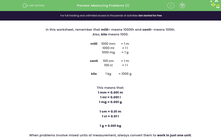# Measuring Problems (1)

In this worksheet, students solve problems involving the calculation and conversion of units of measure, using decimal notation up to three decimal places.Key stage:  KS 2

Curriculum topic:   Measurement

Curriculum subtopic:   Solve Conversion Problems

Difficulty level:#### Worksheet Overview

In this worksheet, remember that milli- means 1000th and centi- means 100th.

Also, kilo means 1000.

 milli 1000 mm = 1 m 1000 ml = 1 l 1000 mg = 1 g centi 100 cm = 1 m 100 cl = 1 l kilo 1 kg = 1000 g

This means that:

1 mm = 0.001 m

1 ml = 0.001 l

1 mg = 0.001 g

1 cm = 0.01 m

1 cl = 0.01 l

1 g = 0.001 kg

When problems involve mixed units of measurement, always convert them to work in just one unit.

Often it is easier to work with the smaller unit of measurement and then convert back at the end if the question asks for the answer in the larger unit of measurement.

Example 1

Jack pours out 2 litres of water into a pan and then removes 32 ml.

How much is left in the pan, in litres?

Work in millilitres.

2000 ml - 32 ml = 1968 ml

Convert back to litres by dividing by 1000.

1.968 l is left in the pan.

Example 2

Ann joins a 52 cm pipe, end to end, to one that is 2.5 m long.

How long are both pipes together in m?

Work in cm.

52 cm + 250 cm = 302 cm

Convert back to m by dividing by 100.

The pipe is 3.02 m long.

### What is EdPlace?

We're your National Curriculum aligned online education content provider helping each child succeed in English, maths and science from year 1 to GCSE. With an EdPlace account you’ll be able to track and measure progress, helping each child achieve their best. We build confidence and attainment by personalising each child’s learning at a level that suits them.

Get started